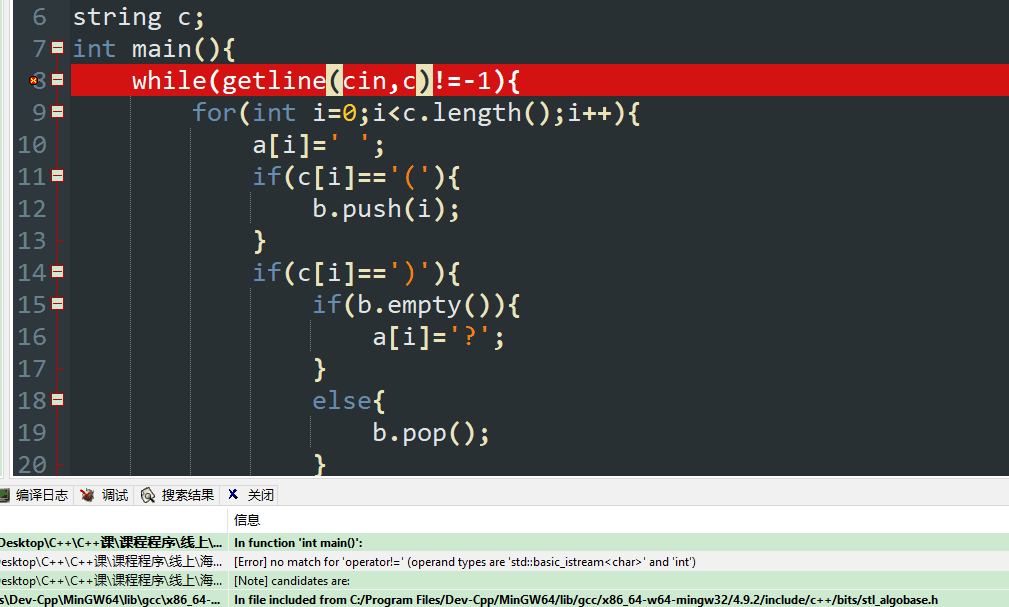﻿ 结题报告-.Net系列-C++-我要学编程_我要学编程是一个面向开发者的知识分享网站

# 结题报告

EOF（-1）仅限用于scanf字符数组，不能用于字符串，否则EOF（-1）不能用于字符串，读入字符串可以这样：

`while(getline(cin,c))//string c`

``` 1 #include <stack>
2 #include <iostream>
3 using namespace std;
4 char a;
5 stack <int> b;
6 string c;
7 int main(){
8     while(getline(cin,c)){
9         for(int i=0;i<c.length();i++){
10             a[i]=' ';
11             if(c[i]=='('){
12                 b.push(i);
13             }
14             if(c[i]==')'){
15                 if(b.empty()){
16                     a[i]='?';
17                 }
18                 else{
19                     b.pop();
20                 }
21             }
22         }
23         while(!b.empty()){
24             int i=b.top();
25             b.pop();
26             a[i]='\$';
27         }
28         a[c.length()]='\0';
29         cout << c << "\n" << a << "\n";
30     }
31     return 0;
32 }```